Courses

# MCQs Of Electrochemistry, Past Year Questions JEE Mains, Class 12, Chemistry

## 35 Questions MCQ Test Class 12 Chemistry 35 Years JEE Mains &Advance Past yr Paper | MCQs Of Electrochemistry, Past Year Questions JEE Mains, Class 12, Chemistry

Description
This mock test of MCQs Of Electrochemistry, Past Year Questions JEE Mains, Class 12, Chemistry for JEE helps you for every JEE entrance exam. This contains 35 Multiple Choice Questions for JEE MCQs Of Electrochemistry, Past Year Questions JEE Mains, Class 12, Chemistry (mcq) to study with solutions a complete question bank. The solved questions answers in this MCQs Of Electrochemistry, Past Year Questions JEE Mains, Class 12, Chemistry quiz give you a good mix of easy questions and tough questions. JEE students definitely take this MCQs Of Electrochemistry, Past Year Questions JEE Mains, Class 12, Chemistry exercise for a better result in the exam. You can find other MCQs Of Electrochemistry, Past Year Questions JEE Mains, Class 12, Chemistry extra questions, long questions & short questions for JEE on EduRev as well by searching above.
QUESTION: 1

### Conductivity (unit Siemen’s S) is directly proportional toarea of the vessel and the concentration of the solution in itand is inversely proportional to the length of the vesselthen the unit of the constant of proportionality is                

Solution:

given :QUESTION: 2

### EMF of a cell in terms of reduction potential of its left andright electrodes is        

Solution:

Ecell = Reduction potential of cathode (right)
– Reduction potential of anode (left)
= Eright – Eleft.

QUESTION: 3

### What will be the emf for the given cell  Pt | H2 (P1) | H+ (aq) | | H2 (P2) | Pt

Solution:

Oxidation half call:-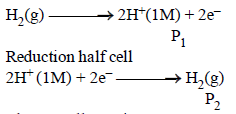The net cell reaction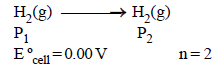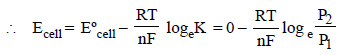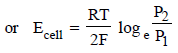QUESTION: 4

Which of the following reaction is possible at anode?

Solution: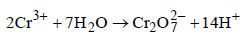O.S. of Cr changes from +3 to +6 by loss of electrons. At anode oxidation takes place.

QUESTION: 5

When the sample of copper with zinc impurity is to be purified by electrolysis, the appropriate electrodes are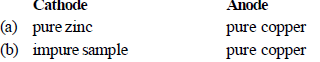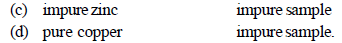Solution:

Pure metal always deposits at cathode

QUESTION: 6

Which of the following is a redox reaction? 

Solution: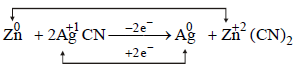QUESTION: 7

For a cell reaction involving a two-electron change, thestandard e.m.f. of the cell is found to be 0.295 V at 25ºC. The equilibrium constant of the reaction at 25ºC will be               [2003

Solution:

The equilibrium constant is related to the standard emf of cell by the expression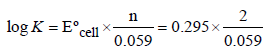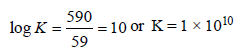QUESTION: 8

Standard reduction electrode potentials of three metals A, B& C are respectively + 0.5 V, – 3.0 V & –1.2 V. The reducingpowers of these metals are 

Solution: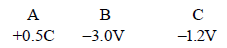NOTE : The higher the negative value of reduction potential, the more is the reducing power.
Hence B > C > A.

QUESTION: 9

When during electrolysis of a solution of AgNO3 9650coulombs of charge pass through the electroplating bath,the mass of silver deposited on the cathode will be

Solution:

When 96500 coulomb of electricity is passed through the electroplating bath the amount of Ag deposited = 108g
∴ when 9650 coulomb of electricity is passed deposited Ag.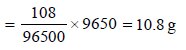QUESTION: 10

For the redox reaction :
Zn(s) +Cu2+ (0.1M)→ Zn2+ (1M) + Cu(s) taking place in a cell,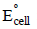is 1.10 volt. Ecell for the cell will  be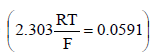Solution: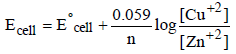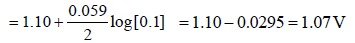QUESTION: 11

Several blocks of magnesium are fixed to the bottom of a ship to                   

Solution:

Magnesium, on account of its lightness, great affinity for oxygen and toughness is used in ship. Being a lighter element, magnesium makes the ship lighter when it is fixed to the bottom of the ship.

QUESTION: 12

In a hydrogen-oxygen fuel cell, combustion of hydrogenoccurs to               

Solution:

In H2 -O2 fuel cell, the combustion of H2 occurs to create potential difference between the two electrodes

QUESTION: 13

Consider the following Eº values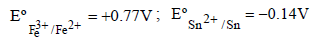Under standard conditions the potential for the reaction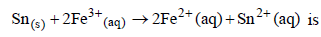Solution:

Fe3+ + e- → Fe2+ΔG° = -1x Fx0.77
Sn2+ + 2e- → Sn(s)ΔG° = -2 x F(-0.14)
for Sn(s) + 2Fe3+ (aq) → 2Fe2+ (aq) +Sn2+ (aq)
∴ Standard potential for the given reaction

∴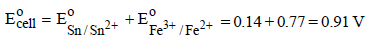QUESTION: 14

The standard e.m.f. of a cell involving one electron changeis found to be 0.591 V at 25ºC. The equilibrium constant ofthe reaction is (F = 96,500 C mol–1; R = 8.314 JK–1 mol–1)                             

Solution: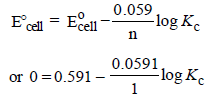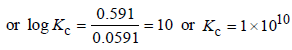QUESTION: 15

The limiting molar conductivities Lº for NaCl, KBr and KClare 126, 152 and 150 S cm2 mol–1 respectively. The Lº forNaBr is 

Solution: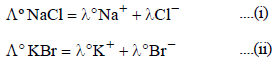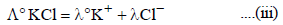operating (i) + (ii) - (iii)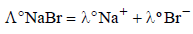= 126 +152 -150 = 128 S cm2 mol-1

QUESTION: 16

In a cell that utilises the reaction  Zn(s) + 2H+ (aq) → Zn2++ H2(g) addition of H2SOto cathode compartment, will          

Solution: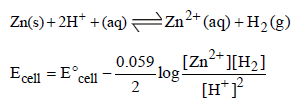QUESTION: 17

The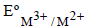values for Cr, Mn, Fe and Co are – 0.41, + 1.57, + 0.77 and + 1.97V respectively. For which one of these
metals the change in oxidation state from +2 to +3 is easiest?               

Solution:

The given values show that Cr has maximum oxidation potental, therefore its oxidation will be easiest. (Change the sign to get the oxidation values)

QUESTION: 18

For a spontaneous reaction the DG, equilibrium constant (K) andwill be respectively 

Solution:

NOTE : For spontaneous reaction DG should be negative. Equilibrium constant should be more than one

(ΔG = – 2.303 RT log Kc, If Kc = 1 then ΔG = 0; If Kc < 1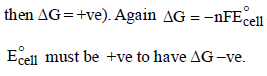QUESTION: 19

The highest electrical conductivity of the following aqueoussolutions is of                     

Solution:

Thus difluoro acetic acid being strongest acid will furnish maximum number of ions showing highest electrical conductivity. The decreasing acidic strength of the carboxylic acids given is difluoro acetic acid > fluoro acetic acid > chloro acitic acid > acetic acid.

QUESTION: 20

Aluminium oxide may be electrolysed at 1000°C to furnishaluminium metal (At. Mass = 27 amu; 1 Faraday = 96,500Coulombs). The cathode reaction is– Al3+ + 3e- → Al° To prepare 5.12 kg of aluminium metal by this method werequire

Solution:

1 mole of e– = 1F = 96500 C
27g of Al is deposited by 3 × 96500 C
5120 g of Al will be deposited by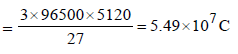QUESTION: 21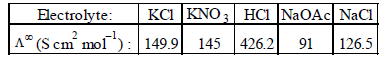Calculate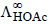using appropriate molar conductances of the electrolytes listed above at infinite dilution in H2O
at 25°C

Solution: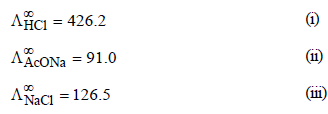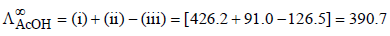QUESTION: 22

Which of the following chemical reactions depict the oxidizing beahviour of H2SO4?

Solution: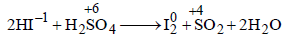reaction oxidation number of S is decreasing from + 6 to +4 hence undergoing reduction and for HI oxidation

Number of I is increasing from –1 to 0 hence
underegoing oxidation therefore H2SO4 is acting as oxidising agent.

QUESTION: 23

The molar conductivities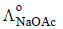and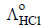at infinite dilution in water at 25ºC are 91.0 and 426.2 S cm2/mol
respectively. To calculate, the additional value required is

Solution: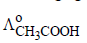is given by the following equation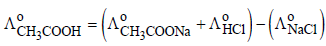Hence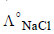is required.

QUESTION: 24

Resistance of a conductivity cell filled with a solution of an electrolyte of concentration 0.1 M is 100 W. The conductivity of this solution is 1.29 S m–1. Resistance of the same cell when filled with 0.2 M of the same solution is 520 W. The molar conductivity of 0.2 M solution of electrolyte will be                            

Solution: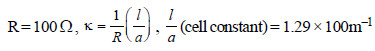Given, R = 520Ω, C = 0.2 M, μ (molar conductivity) = ?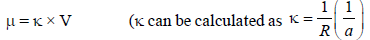now cell constant is known.)

Hence,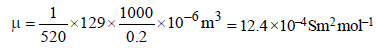QUESTION: 25

The equivalent conductances of two strong electrolytes at infinite dilution in H2O (where ions move freely through a
solution) at 25°C are given below :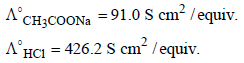What additional information/ quantity one needs to calculate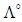of an aqueous solution of acetic acid?

Solution:

NOTE : According to Kohlrausch’s law, molar
conductivity of weak electrolyte acetic acid (CH3COOH) can be calculated as follows: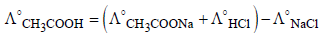∴ Value ofshould also be known for
calculating value of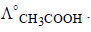QUESTION: 26

The cell, Zn | Zn2+ (1 M) || Cu2+ (1 M) | Cu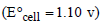was allowed to be completely discharged at 298 K. The relative
concentration of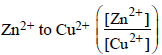is         

Solution:

Ecell = 0; when cell is completely discharged.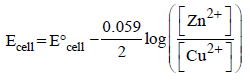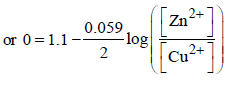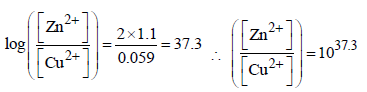QUESTION: 27

Given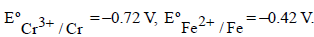The potential for the cell Cr|Cr3+ (0.1M)|| Fe2+ (0.01 M)| Fe is         

Solution:

From the given representation of the cell, Ecell can be found as follows.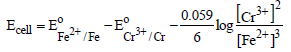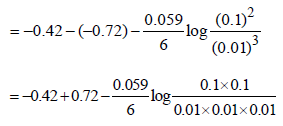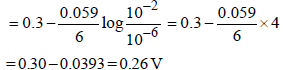Hence option (d) is correct answer.

QUESTION: 28

In a fuel cell methanol is used as fuel and oxygen gas is used as an oxidizer. The reaction is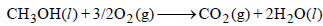At 298 K standard Gibb’s energies of formation for CH3OH(l), H2O(l) and and CO2 (g) are –166.2,  –237.2 and –394.4 kJ
mol–1 respectively. If standard enthalpy of combustion of methonal is – 726 kJ mol–1, efficiency of the fuel cell will be:



Solution: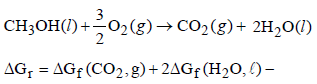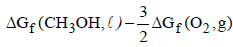= – 394.4 + 2 (–237.2) – (–166.2) – 0
= – 394.4 – 474.4 + 166.2 = – 702.6 k J
% efficiency =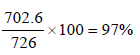QUESTION: 29

Given: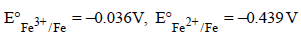The value of standard electrode potential for the change, Fe3+ (aq) + e →Fe2+ (aq) will be:



Solution:

Given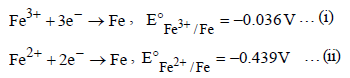we have to calculate

Fe3+ + e → Fe2+ … (iii)
As we know that ΔG = –nFE
Thus for reaction (iii)

ΔG = ΔG1 - ΔG ; – nFE° = – nFE1 – (–nFE2)
–nFE° = nFE2 – nFE1
–1FE° = 2× 0.439F – 3 × 0.036 F
–1 FE° = 0.770 F ∴ E° = – 0.770V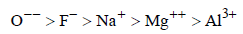QUESTION: 30

The Gibbs energy for the decomposition of Al2O3 at 500°C is as follows :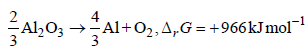The potential difference needed for electrolytic reductionof Al2O3 at 500°C is at least                      

Solution: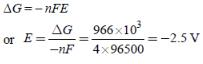QUESTION: 31

The correct order of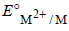values with negative sign for the four successive elements Cr, Mn, Fe and Co is 

Solution:

The value of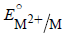for given metal ions are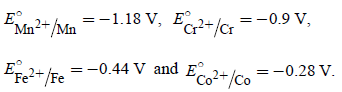The correct order ofvalues without
considering negative sign would be

Mn2+ > Cr2+ > Fe2+ > Co2+.

QUESTION: 32

The reduction potential of hydrogen half-cell will be negative if :



Solution: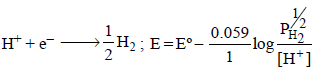Now if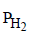=  2 atm and [H+] = 1M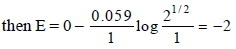QUESTION: 33

The standard reduction potentials for Zn2+/Zn, Ni2+/Ni and Fe2+/Fe are –0.76,–0.23 and –0.44 V respectively.
The reaction X+Y2+ →X2++Y will be spontaneous when :

Solution:

For a spontaneous reaction DG must be –ve
Since ΔG = – nFE°
Hence for ΔG to be -ve ΔE° has to be positive. Which is possible when X = Zn, Y = Ni
Zn + Ni++ →  Zn++ + Ni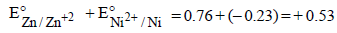QUESTION: 34

Given :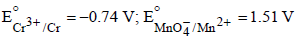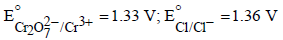Based on the data given above, strongest oxidising agentwill be : [JEE M 2013]

Solution:

higher the value of standard reduction potential
stronger will be the oxidising agent, hence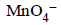is  the strongest oxidising agent.

QUESTION: 35

Resistance of 0.2 M solution of an electrolyte is 50 Ω.The specific conductance of the solution is 1.4 S m–1.The resistance of 0.5 M solution of the same electrolyte is 280 Ω. The molar conductivity of 0.5 M solution of the electrolyte in S m2 mol–1 is:

[JEE M 2014]

Solution:

Given for 0.2 M solution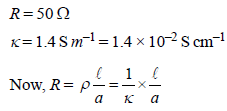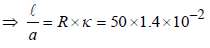For 0.5 M solution
R = 280 Ω; k = ?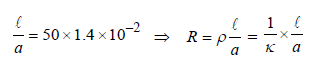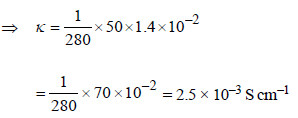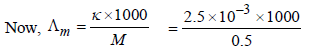= 5 S cm2 mol–1 = 5 × 10–4 S m2 mol–1